Courses
Courses for Kids
Free study material
Offline Centres
More

# Hinge Force - JEE Important TopicLast updated date: 28th Nov 2023
Total views: 21.6k
Views today: 0.21k## What is Hinge Force?

A hinge is a mechanical bearing that interconnects two rigid bodies, usually authorising only a restricted angle of revolving between them. Two rigid bodies are connected by an ideal hinge revolve corresponding to each other about a fixed axis of rotation. All other translations or rotations are averted. This is the reason a hinge has only one degree of freedom. Hinges may be constructed with malleable material or from moving components. In biological terms, many linkages or joints are hinges, like the elbow joint. Let us have a detailed discussion about what is hinge force, how to find hinge force and the direction of hinge force in this article.

## Derivation of Hinge Force Formula

Consider a rigid isosceles triangle frame constructed by three uniform thin rods (mass = m & length = l) that is free to revolve evenly in the vertical plane. The frame is hinged at one of its vertices H.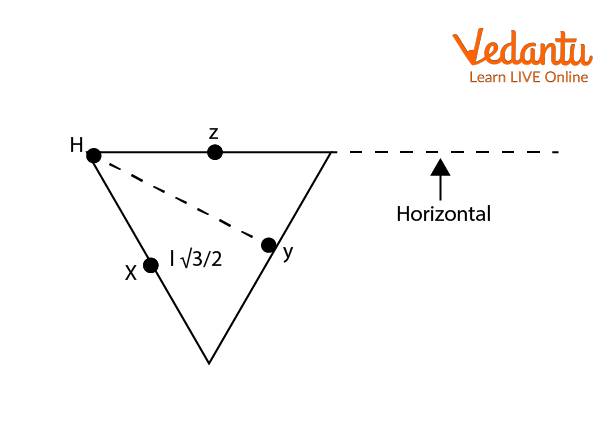Impulsive Hinge

The frame is free from rest from the position that is illustrated in the above figure. The individual weights act downwards at points x, y, and z as shown in the figure which is w = mg

The moment concerning the x-axis is $M_{x}=\dfrac{1}{2} m gl$

The moment concerning the y-axis is $M_{y}=\dfrac{1}{2} m g l \sin 30^{0}$

The moment concerning the z-axis is $M_{z}=\dfrac{\sqrt{3}}{2} m g l \cos 30^{0}$

All the three torques applied by the weights are in a clockwise direction about the point H.

$\tau_{\text {total }}=m g\left(\dfrac{1}{2}+\dfrac{1}{2} \sin 30^{0}+\dfrac{\sqrt{3}}{2} l \cos 30^{\circ}\right)$

$\tau_{\text {total }}= m g l\left(\dfrac{1}{2}+\dfrac{1}{2} \times \dfrac{1}{2}+\dfrac{\sqrt{3}}{2} \times \dfrac{\sqrt{3}}{2}\right)$

$\tau_{\text {total }} =\dfrac{3}{2} m g l$

Now, the moment of inertia (MI) will be

MI of $I_{H A}=\dfrac{m l^{2}}{3}$

MI of $I_{H B}=\dfrac{m l^{2}}{3}$

MI of $I_{A B}=\dfrac{m l^{2}}{12}+m\left(\dfrac{\sqrt{3}}{2} l\right)^{2}=\dfrac{5 m l^{2}}{6}$

So, the total moment of inertia will be

$I_{\text {total }}=I_{H A}+I_{H B}+I_{A B}=\dfrac{m l^{2}}{3}+\dfrac{m l^{2}}{3}+\dfrac{5 m l^{2}}{6}=\dfrac{3}{2} m l^{2}$

Equating the $\tau_{total}$ to the Itotal which is multiplied by $\omega$ we get:

\begin{align} &\dfrac{3}{2} m g l=\dfrac{3}{2} m l^{2} \omega \\ \\ &\Rightarrow \omega=\dfrac{g}{l} \end{align}

Now for the hinge force, we have

$N_{1}=3 m g \cos 60^{\circ}=\dfrac{3}{2} m g$

$3 m g \sin 60^{\circ}-N_{2}=3 m \times \dfrac{g}{l} \times \dfrac{1}{\sqrt{3}}$

$\Rightarrow N_{2}=\dfrac{\sqrt{3}}{2} m g$

So, the formula of hinge force will be $\sqrt{N_{1}^{2}+N_{2}^{2}}=\sqrt{3 m g}$

## Hinge Force Diagram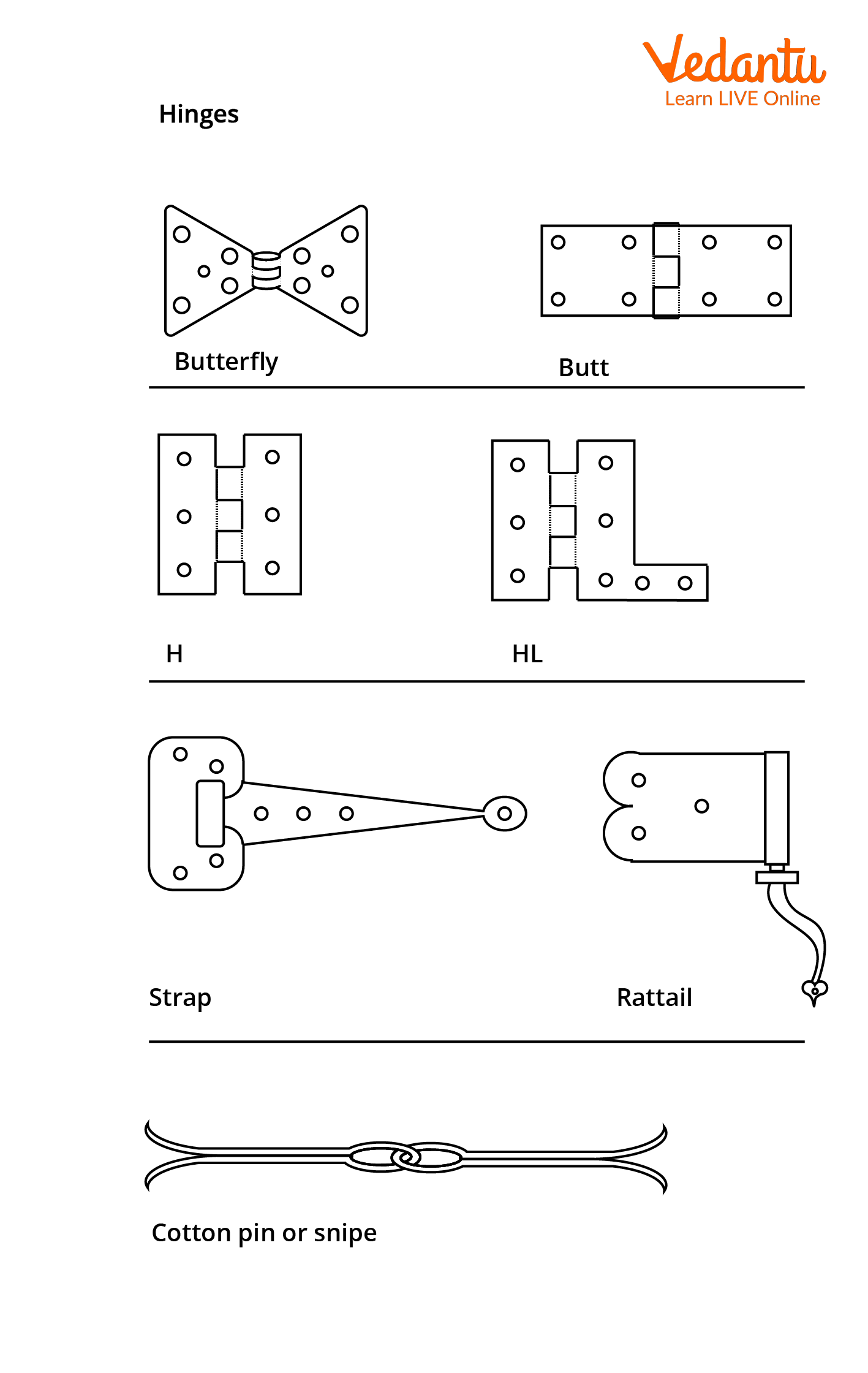Various Hinge Diagrams

In this hinge diagram, there are many hinge arrangements. Let us talk about all these in a very simple manner.

### Butterfly Hinge

A butterfly hinge is a type of strap hinge which promotes leaf plates that are arranged in a decorative manner and favours the wings of a butterfly. It is invariably mounted on the surface. Frequently, it is constructed with brass or any other decorative metal and is mostly used on ornate boxes or attractive cabinets. It is also called the parliament hinge.

### Butt Hinge

Butt hinges are also known as mortise hinges. The butt hinge is mostly used in a couple of doors. It is constructed by two identical leaves of metal interconnected by a central pin and barrel system. One leaf of the butt hinge is mortised or sunken towards the door and the other is attached to the pillar. When duly positioned, the two leaves of a butt hinge should perch flush against each other, which permits the door to perch perfectly flush with the pillar.

### H-Hinge

These types of barrel hinges are arranged like an H and are extensively used on flush-mounted doors. Small H hinges of measurement 3–4 inches or 76–102 mm are used in cabinet hinges, larger hinges of about  6–7 inches or 150–180 mm, on the other hand, are used for passage doors or cupboards doors.

### HL Hinge

These are common for cupboard doors, room doors, and passage doors.

### Strap Hinge

A strap hinge is a hinge which has a long flutter by which it is buckled to the surface of a door and the abutting wall. It is compared with a butt hinge.

### Rattail Hinge

A rattail hinge is a flexible type of hinge in which the pin is enlarged so that it can be fastened to the sheathing of a door.

### Snipe Hinge

Early American pilgrim fixture hinges containing a couple of half-round iron laces double back like cotter pins, connected by the eyes, and grapple into the logs at the serrated outer ends.

Let us now understand what is meant by hinge reaction.

## Hinge Reaction

The determination of hinge reaction is important to simplify the analysis that each support utilises on the trap door across each axis of a Cartesian coordinate system. Each reaction is presumed to be acting in a positive direction.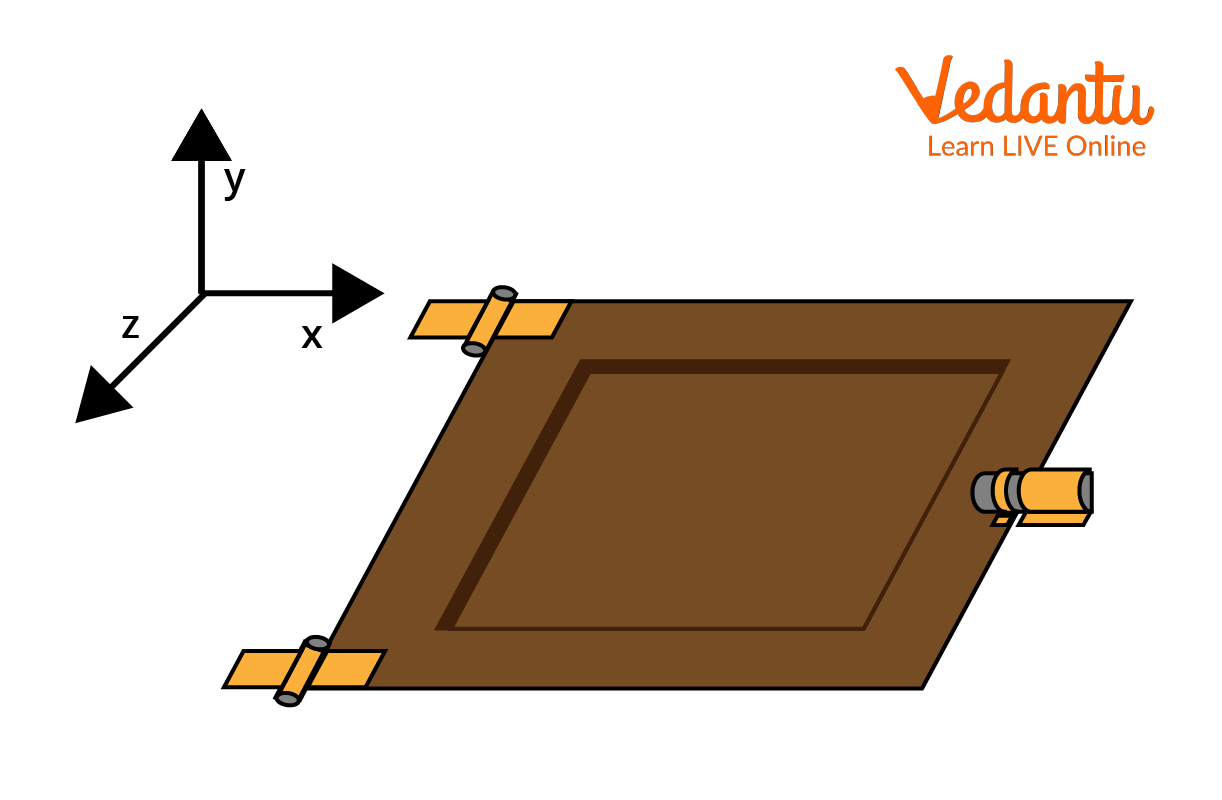Coordinate System

### Support 1:

The first support emerged to be a standard hinge at a quick glance. Upon further examination, it is clear that this support is actually a bearing with a circular shaft that can glide along the z-axis (which standard hinges cannot). This type of support has two unknown reaction forces and two unknown reaction moments along the coordinate axes that are perpendicular to the axis of the bearing. However, there are no reaction forces or moments along the axis of the bearing. Hence, the bearing can both translate and revolve along this axis.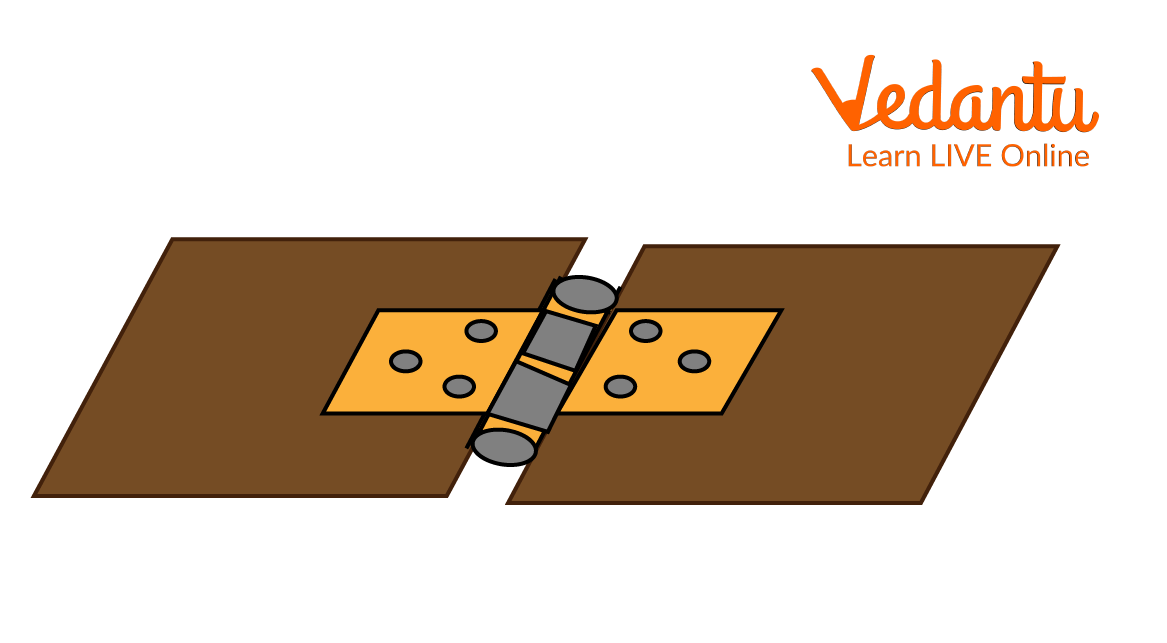Support 1 of a Trap Door

This is the image of support 1. Now let us see the image of how the reaction would be at support 1.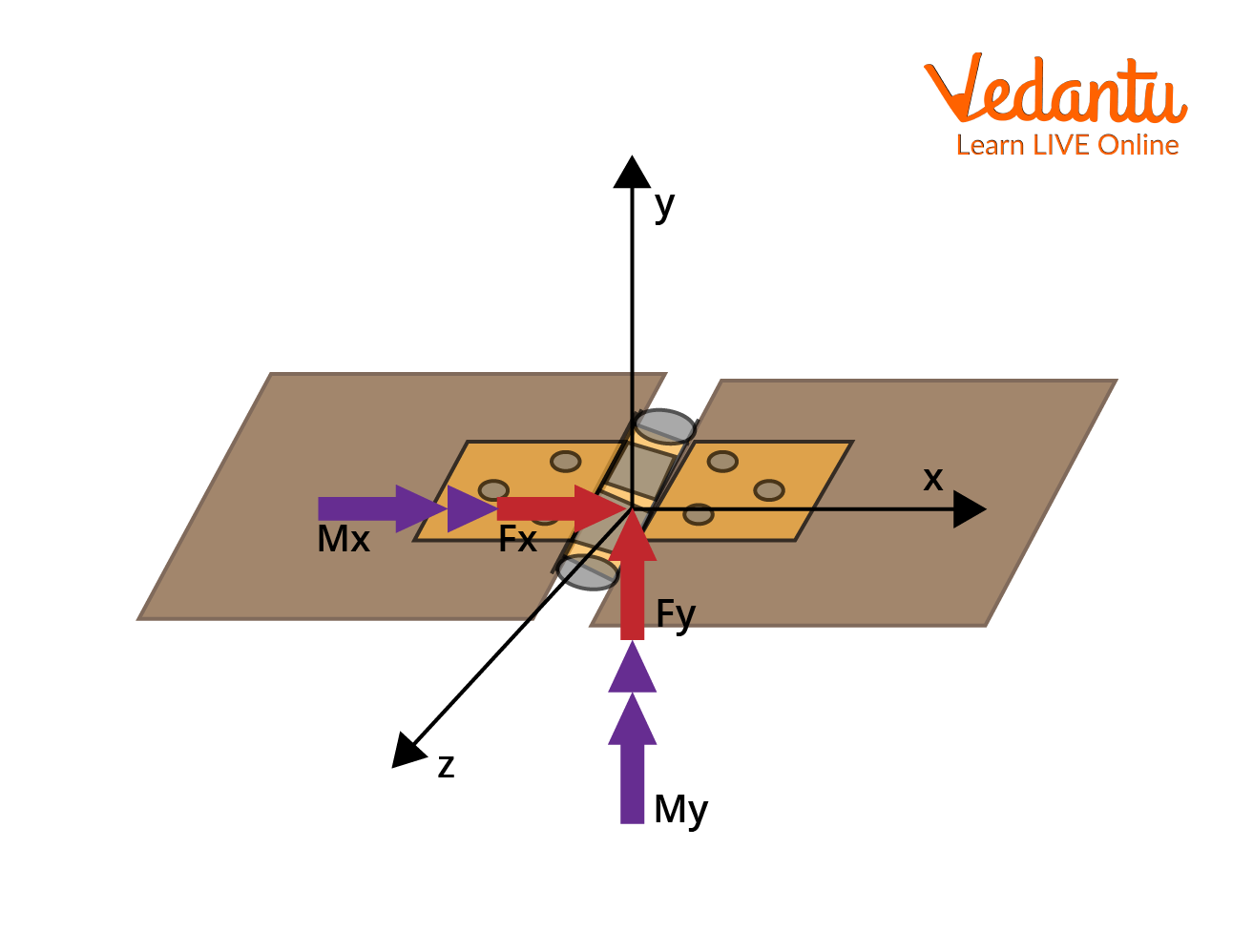Reaction at Support 1

### Support 2:

The second support is a standard hinge. There are two unknown reaction forces and two unknown reaction moments along the coordinate axes that are right-angled to the axis of the hinge. There is also a reaction force along the axis of the hinge (i.e. does not glide along the z-axis). However,  there is no reaction moment along the axis of the hinge. Hence, the hinge may only revolve about this axis.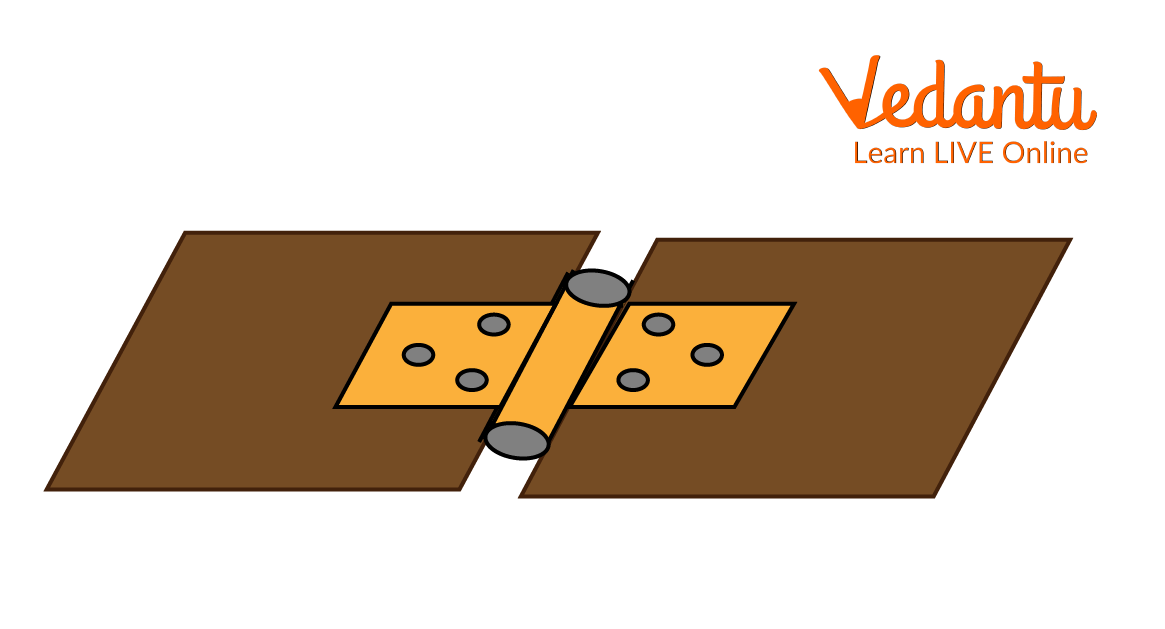Support 2 Diagram of the Trap Door

This is the image of support 2. Now let us see the image of how the reaction would be at support 2.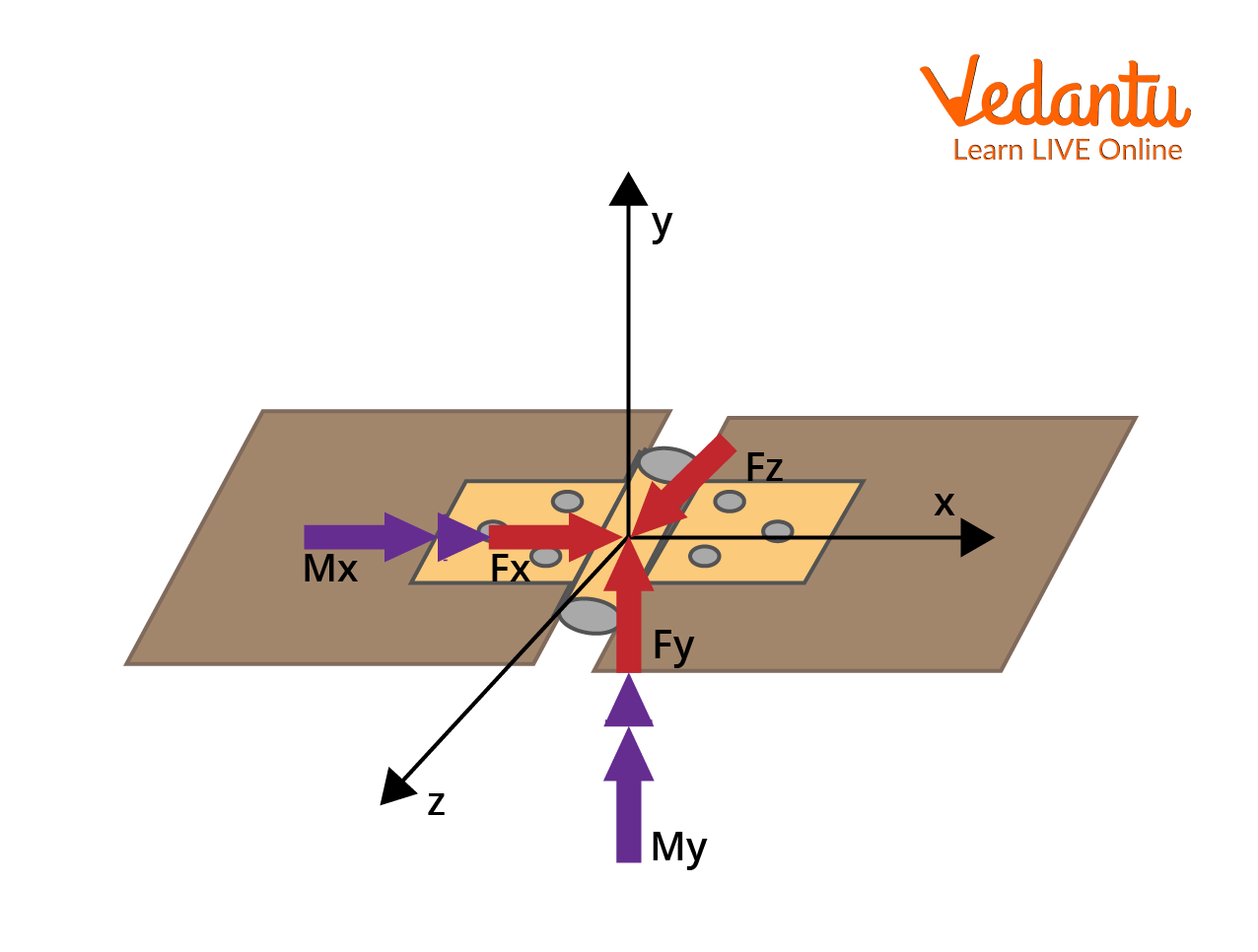Reactions in support 2

This figure is the reaction at support 2 and now let us see support 3.

### Support 3:

The third support is the bolt. This support does not allow any rotation around any axis. Similar to support 2, there are also two unknown reaction forces and two unknown reaction moments along the coordinate axes. However, they are perpendicular to the axis of bearing. There is also a reaction moment along the axis of the bolt. Hence, the bolt only allows translation along the axis of the bolt.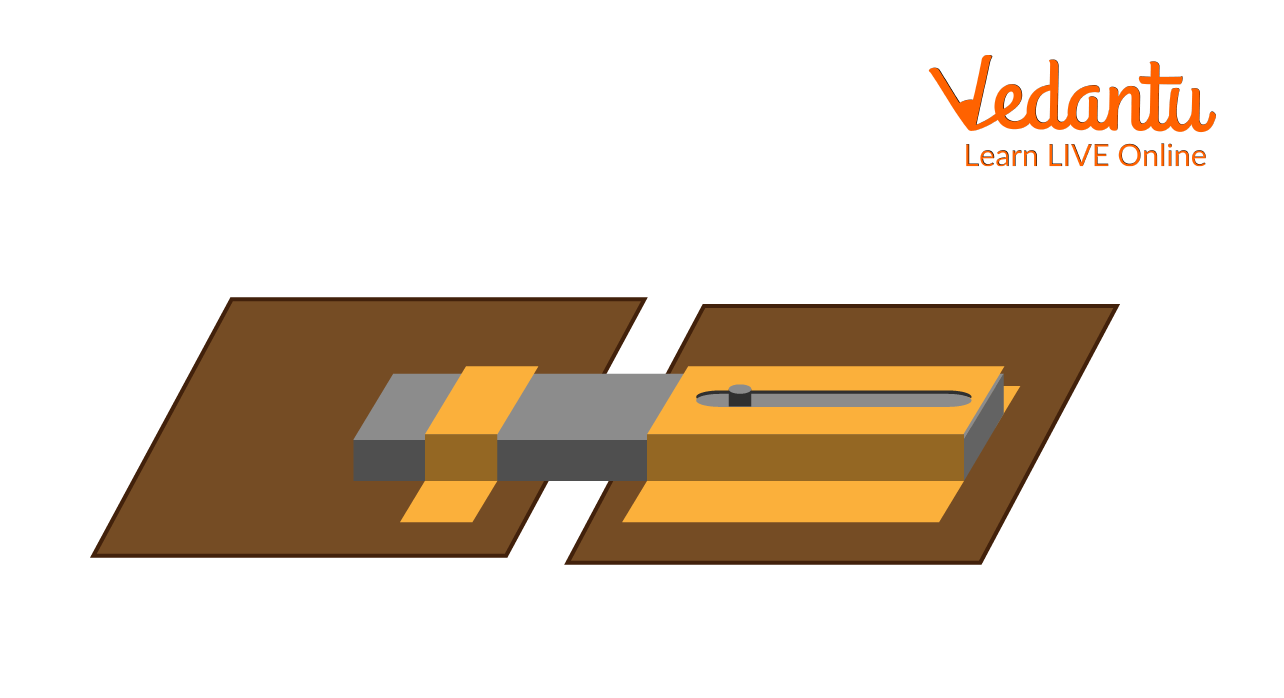Support 3 Diagram

This is the image of support 3. Now let us see the image of how the reaction would be at support 3.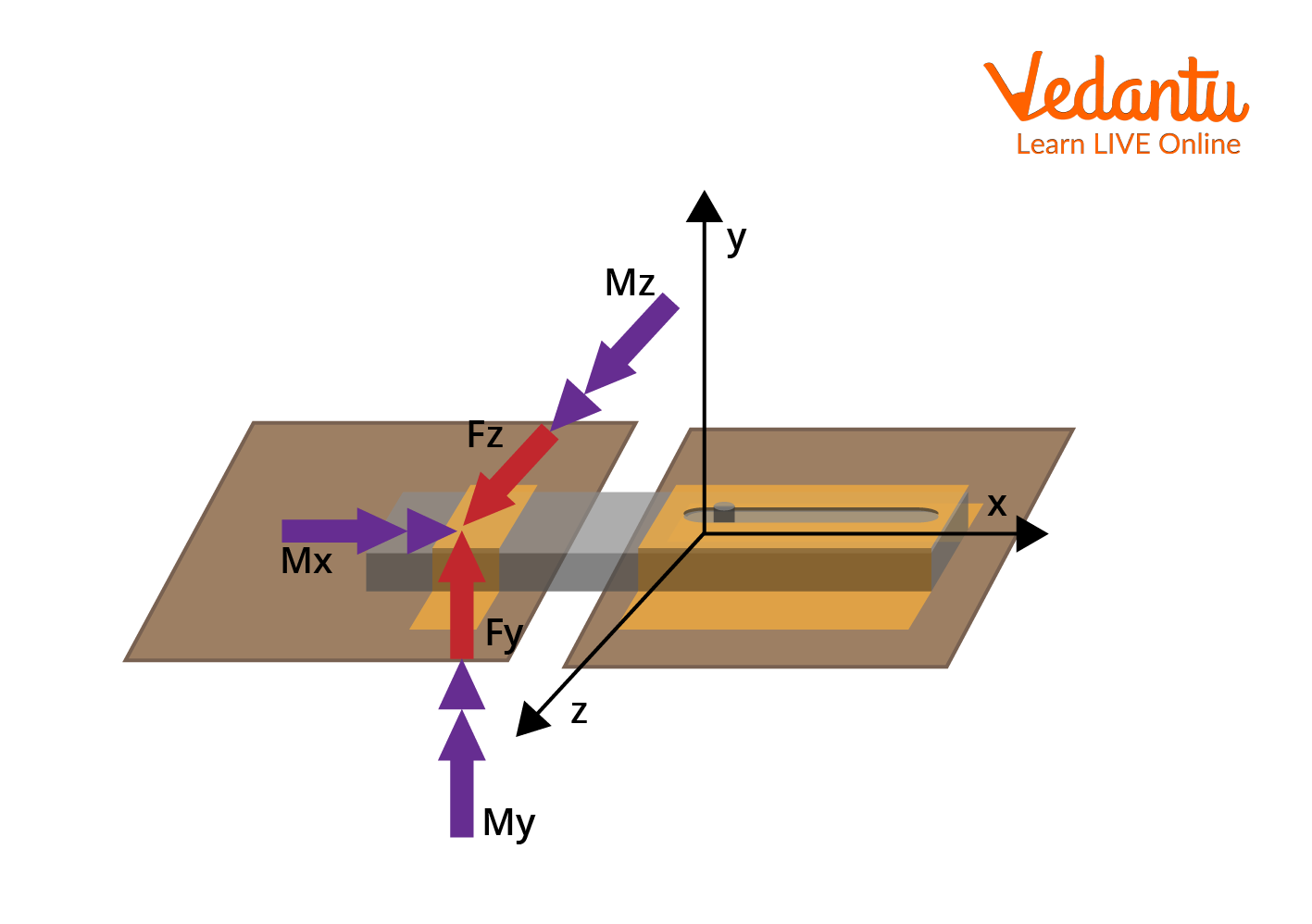Reaction in Support 3

This figure is the reaction at support 3. Now let us understand the direction of hinge force with the help of a diagram.

## Direction of Hinge Force

The understanding of the direction of a hinge force can be simply facilitated by the diagram shown below.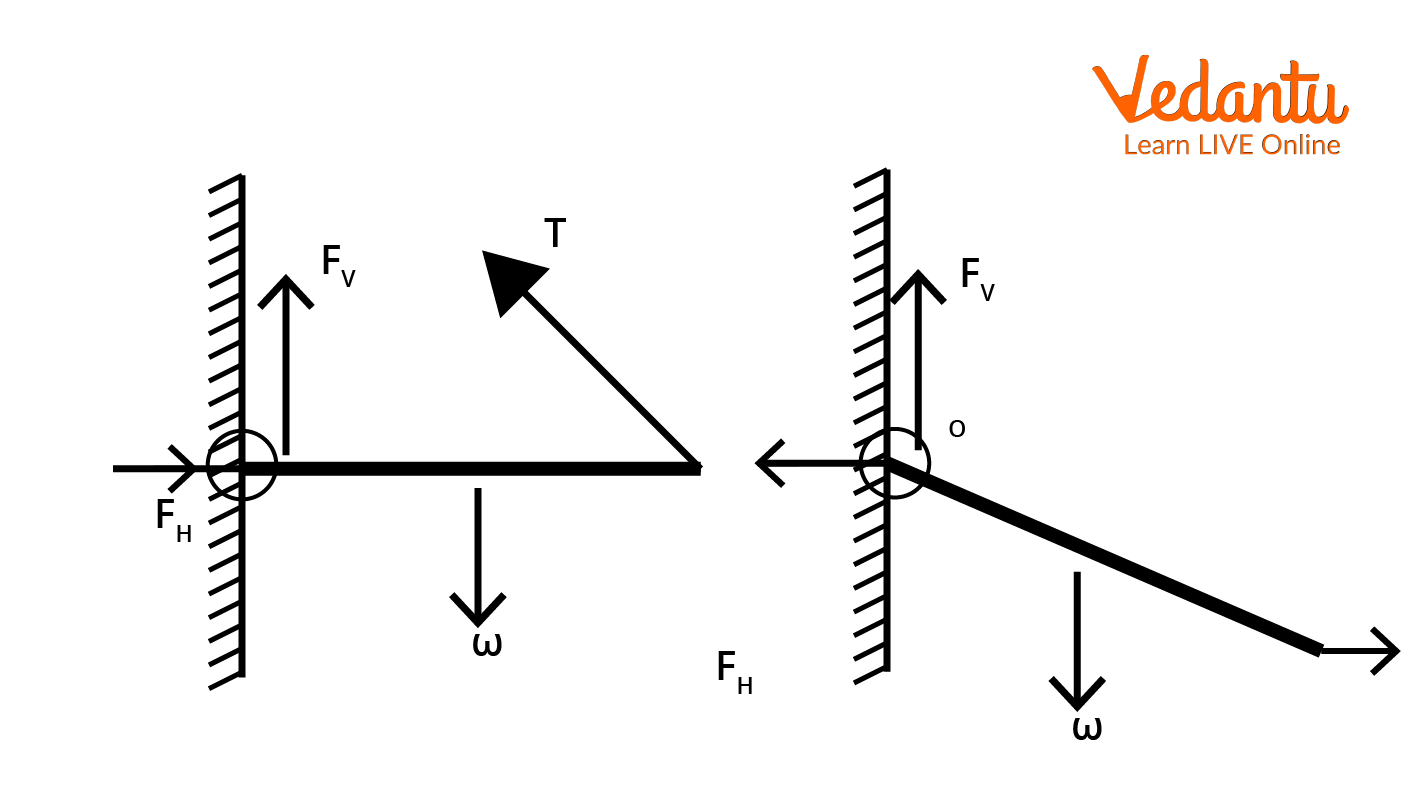Direction of a Hinged Force

Since the bar in the 1st diagram is in equilibrium along the horizontal, the reaction at the hinge must be opposite to the horizontal component of applied force at the free end, as we understood earlier by the reaction forces acting on a hinged end. However, if numerous forces are acting, we can presume a general situation with a horizontal and vertical component. Applying equilibrium conditions will tell us if the assumed direction was right or wrong. Positive answers infer the correct assumption and negative infers the opposite.

In the second diagram, the only non-hinge force with a horizontal element is the force that is labelled as T in the diagram. Consequently, the horizontal element of the hinge force is required to be equal and opposite to the horizontal element of T.

## Conclusion

The hinge force is important in engineering mechanics to illustrate the free body diagram of a frame which is hinged. The article clearly describes the different types of hinge joints and a brief idea about all these types along with the different reaction forces which are acting on a hinged body. Through the different support arrangements, we can now understand the different reaction moments which are along the coordinate axis. These reaction moments are perpendicular as well as parallel according to the support arrangements. We can also gain a descriptive idea about the hinge force from this article.

## FAQs on Hinge Force - JEE Important Topic

1. Is there any normal force acting on the hinge?

By definition, normal force is always right-angled to the common surface at which the objects make a contact. If there is no hinge, this surface will be the wall. There can also be a frictional force in which case the reaction force at the wall can point in any orientation away from the wall. With a hinge, the normal force between the hinge and the rod can point in any orientation, even towards the wall or away from the wall.

2. How much weight can a hinge hold?

The hinges which perform the standard duty for the low or the medium-frequency doors can hold the weight up to 200 lb, without frame or door reinforcement. However, the hinges that perform a heavy duty for high-frequency doors can hold the weight up to 200 lb. For doors with a medium frequency, the hinges can hold up to 400 lb, both without frame or door reinforcement. Whereas, for low-frequency doors, the hinges used can hold up to 600 lb weight.